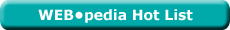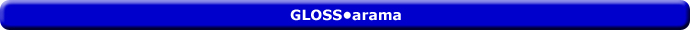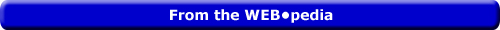Tuesday  June 28, 2022
 AmosWEB means Economics with a Touch of Whimsy!AD CURVE: The aggregate demand curve, which is a graphical representation of the relation between aggregate expenditures on real production and the price level, holding all ceteris paribus aggregate demand determinants constant. The aggregate demand, or AD, curve is one side of the graphical presentation of the aggregate market. The other side is occupied by the aggregate supply curve (which is actually two curves, the long-run aggregate supply curve and the short-run aggregate supply curve). The negative slope of the aggregate demand curve captures the inverse relation between aggregate expenditures on real production and the price level. This negative slope is attributable to the interest-rate effect, real-balance effect, and net-export effect.Most Viewed (Number) Visit the WEB*pediaLONG-RUN AVERAGE COST CURVE: A curve depicting the per unit cost of producing a good or service in the long run when all inputs are variable. The long-run average cost curve (usually abbreviated LRAC) can be derived in two ways. On is to plot long-run average cost, which is, long-run total cost divided by the quantity of output produced. at different output levels. The more common method, however, is as an envelope of an infinite number of short-run average total cost curves. Such an envelope is base on identifying the point on each short-run average total cost curve that provides the lowest possible average cost for each quantity of output. The long-run average cost curve is U-shaped, reflecting economies of scale (or increasing returns to scale) when negatively-sloped and diseconomies of scale (or decreasing returns to scale) when positively sloped. The minimum point (or range) on the LRAC curve is the minimum efficient scale.Recommended Citation:

LONG-RUN AVERAGE COST CURVE, AmosWEB GLOSS*arama, http://www.AmosWEB.com, AmosWEB LLC, 2000-2022. [Accessed: June 28, 2022].

Search Again?MARGINAL COST AND LAW OF DIMINISHING MARGINAL RETURNS

Decreasing then increasing marginal cost, reflected by a U-shaped marginal cost curve, is the result of increasing then decreasing marginal returns. In particular the decreasing marginal returns is caused by the law of diminishing marginal returns. As such, the law of diminishing marginal returns affects not only the short-run production of a firm but also the cost of short-run production. This translates into a positively-sloped supply curve for profit-maximizing competitive firms.YELLOW CHIPPEROON[What's This?] Today, you are likely to spend a great deal of time strolling around a discount warehouse buying club trying to buy either a coffee cup commemorating last Friday (you know why) or a wall poster commemorating the first day of spring. Be on the lookout for rusty deck screws.Your Complete ScopePost WWI induced hyperinflation in German in the early 1900s raised prices by 726 million times from 1918 to 1923."I do not believe in a fate that will fall on us no matter what we do. I do believe in a fate that will fall on us if we do nothing. "-- Ronald Reagan, 40th US presidentMPCMarginal Propensity to ConsumeA PEDestrian's Guide Xtra CreditTell us what you think about AmosWEB. Like what you see? Have suggestions for improvements? Let us know. Click the User Feedback link.| | | | | | | | | | |
| | | |

Thanks for visiting AmosWEB